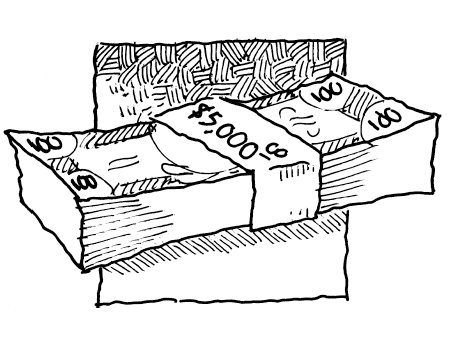### Home > PC3 > Chapter 13 > Lesson 13.1.3 > Problem13-40

13-40.Most investments receive compound interest as opposed to simple interest. This allows an investment to earn interest on the interest already received. Miranda plans to invest $5000$. Calculate the value of the investment after one year, given the following rates:

$A=P\left(1+\frac{r}{n}\right)^{nt}$

1. Annual interest of $12\%$ compounded monthly.

2. Annual interest of $8\%$ compounded quarterly.

3. Annual interest of $5.2\%$ compounded weekly.

4. Annual interest of $7.3\%$ compounded daily.